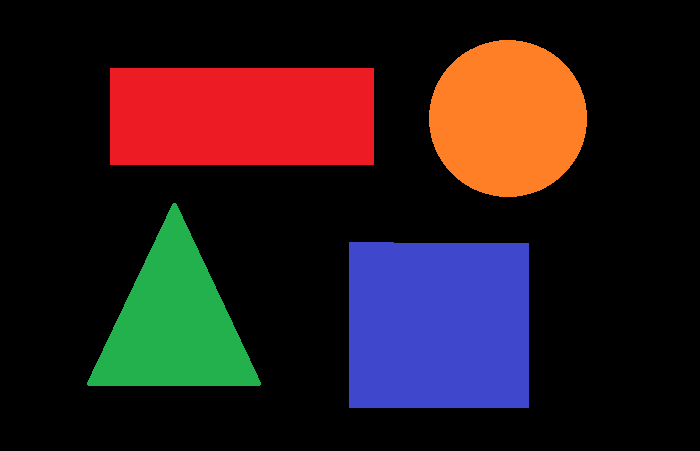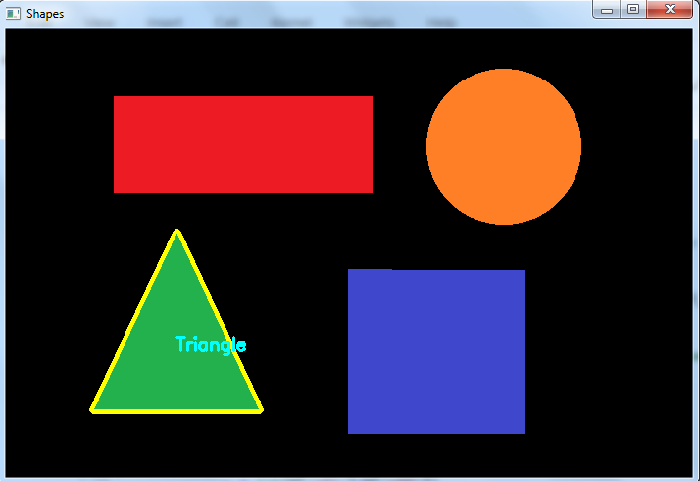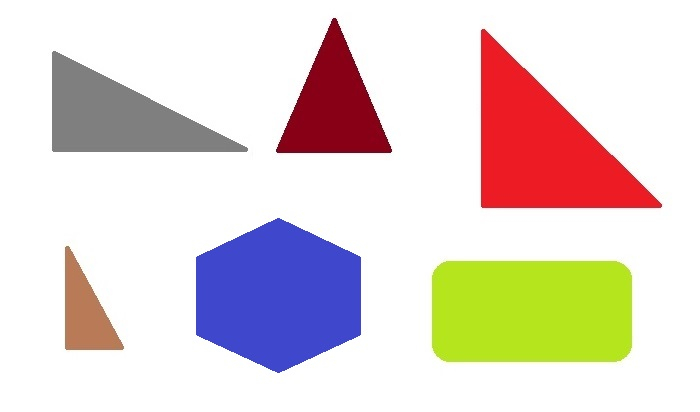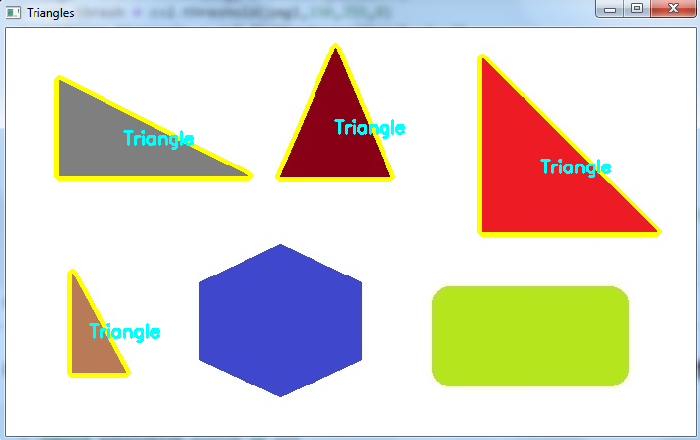# How to detect a triangle in an image using OpenCV Python?

To detect a triangle in an image, we first detect all the contours in the image. Then we loop over all the contours. Find the approximate contour for each of the contours. If the number of vertex points in the approximate contour is 3, then draw the contour and set it as a triangle. See the below pseudocode.

for cnt in contours:
approx = cv2.approxPolyDP()
if len(approx) == 3:
cv2.drawContours()
cv2.putText("Triangle")


## Steps

You can use the following steps to detect a triangle in the input image −

Import the required library. In all the following Python examples, the required Python library is OpenCV. Make sure you have already installed it.

import cv2


img = cv2.imread('approx1.png')
gray = cv2.cvtColor(img, cv2.COLOR_BGR2GRAY)


Apply thresholding on the grayscale image to create a binary image. Adjust the second parameter to get a better contour detection.

ret,thresh = cv2.threshold(gray,50,255,0)


Find the contours in the image using cv2.findContours() function.

contours, _ = cv2.findContours(thresh, cv2.RETR_TREE, cv2.CHAIN_APPROX_SIMPLE)


Select a contour (say first contour) cnt from the lists of contours. Or Loop over all the contours.

Compute the approximate contour points for each contour cnt using cv2.approxPolyDP() function.

approx = cv2.approxPolyDP(cnt,epsilon,True)


If the vertex points in the approximate contour approx is 3, then draw the contour on the image and set it a triangle.

Display the image with drawn contours and approximate contours on it.

cv2.imshow("Shapes", img)
cv2.waitKey(0)
cv2.destroyAllWindows()


Let's look at some examples for a better understanding.

## Example 1

In the Python code below, we detect the triangle in the input image.

# import required libraries
import cv2

# convert the image to grayscale
gray = cv2.cvtColor(img, cv2.COLOR_BGR2GRAY)

# apply thresholding to convert the grayscale image to a binary image
ret,thresh = cv2.threshold(gray,50,255,0)

# find the contours
contours,hierarchy = cv2.findContours(thresh, cv2.RETR_TREE,cv2.CHAIN_APPROX_SIMPLE)
print("Number of contours detected:",len(contours))

for cnt in contours:
approx = cv2.approxPolyDP(cnt, 0.01*cv2.arcLength(cnt, True), True)
if len(approx) == 3:
img = cv2.drawContours(img, [cnt], -1, (0,255,255), 3)

# compute the center of mass of the triangle
M = cv2.moments(cnt)
if M['m00'] != 0.0:
x = int(M['m10']/M['m00'])
y = int(M['m01']/M['m00'])
cv2.putText(img, 'Triangle', (x, y), cv2.FONT_HERSHEY_SIMPLEX, 0.6, (255, 255, 0), 2)

cv2.imshow("Shapes", img)
cv2.waitKey(0)
cv2.destroyAllWindows()


We will use the following image as the Input File in the above program code −## Output

When you execute the above code, it will produce the following output on the console.

Number of contours detected: 4


And we get the following window, showing the outputIn the above output image, one triangle is detected.

## Example 2

In this example, we will show how you can detect multiple triangles in an image.

import cv2
import numpy as np

img1 = cv2.cvtColor(img, cv2.COLOR_BGR2GRAY)

ret,thresh = cv2.threshold(img1,150,255,0)
contours,hierarchy = cv2.findContours(thresh, 1, 2)
print("Number of contours detected:",len(contours))

for cnt in contours:
approx = cv2.approxPolyDP(cnt, 0.1*cv2.arcLength(cnt, True), True)
if len(approx) == 3:
img = cv2.drawContours(img, [cnt], -1, (0,255,255), 3)
M = cv2.moments(cnt)
if M['m00'] != 0.0:
x = int(M['m10']/M['m00'])
y = int(M['m01']/M['m00'])
cv2.putText(img, 'Triangle', (x, y), cv2.FONT_HERSHEY_SIMPLEX, 0.6, (255, 255, 0), 2)

cv2.imshow("Triangles", img)
cv2.waitKey(0)
cv2.destroyAllWindows()


We will use the following image as the Input File in this program −## Output

When you execute the above code, it will produce the following output on the console −

Number of contours detected: 6


And we get the following window, showing the outputIn the above output image, we detected four triangles.